\u200E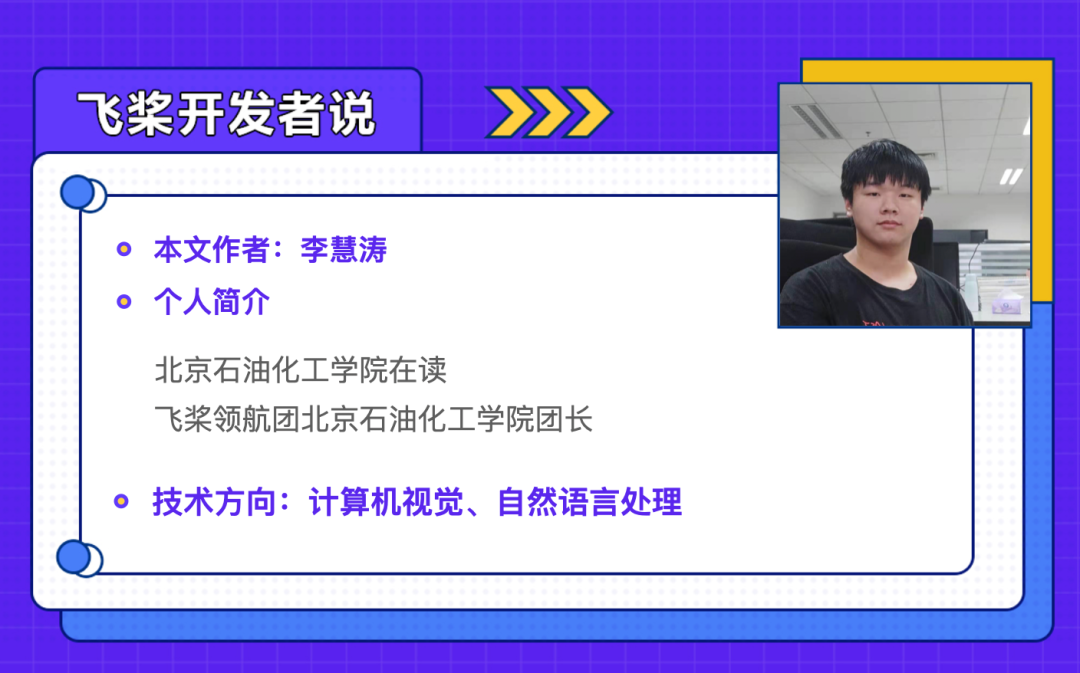CV Transformer的时代已经到来！

https://aistudio.baidu.com/aistudio/projectdetail/2265776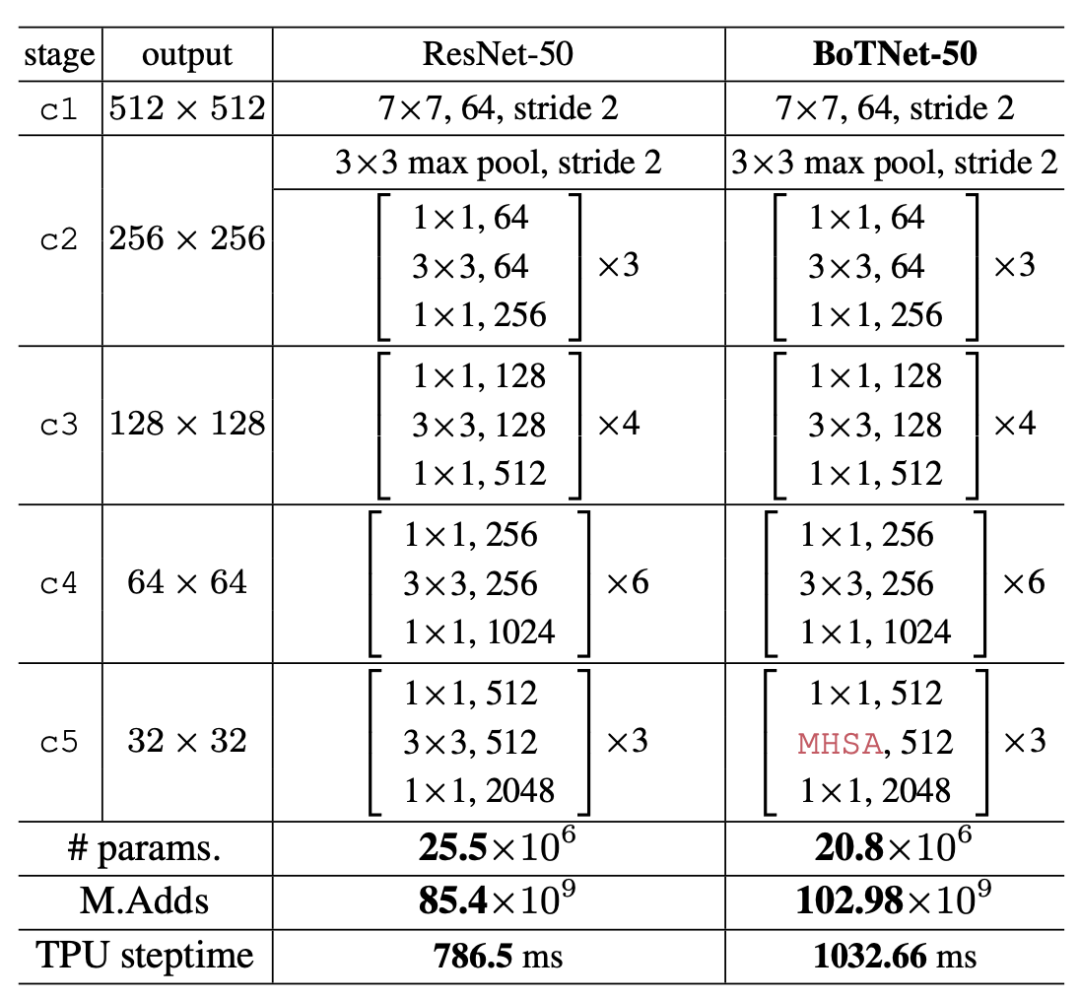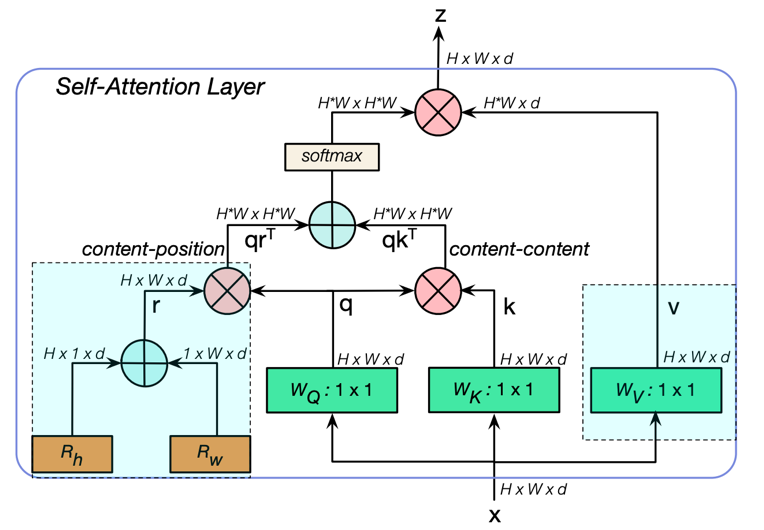BoTNet块中使用的多头自注意 (MHSA) 层。all2all attention是在 2D 特征图上执行的，其中高度和宽度的相对位置编码分别为 Rh 和 Rw。logits attention是 qkT + qrT，其中 q; k; r 分别代表查询、键和位置编码。十 和 X 分别代表逐元素求和和矩阵乘法，而 1x1 代表逐点卷积。蓝色的部分分别代表position encodings 和 value projection。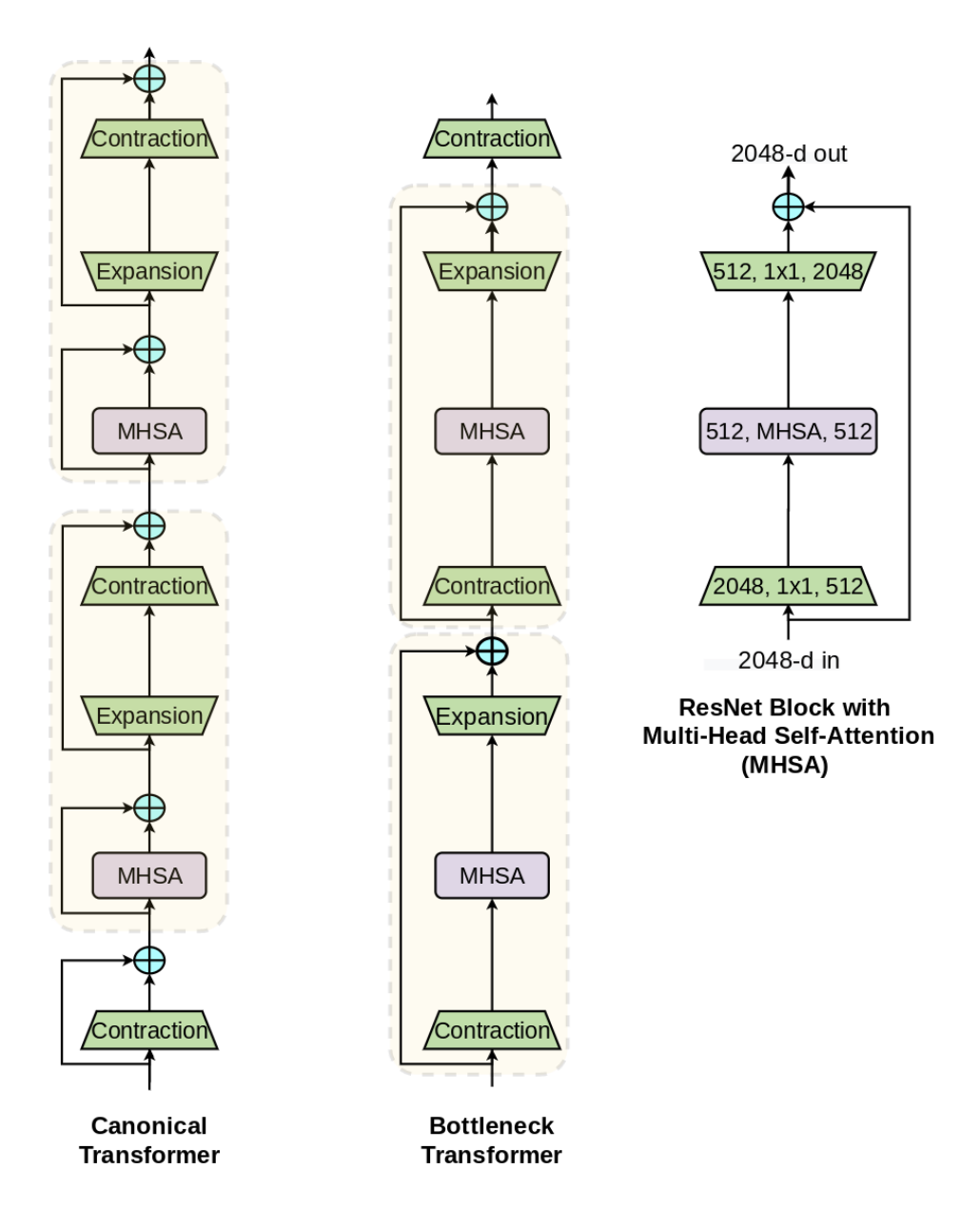``def get_n_params(model):    pp=0    for p in list(model.parameters()):        nn=1        for s in list(p.size()):            nn = nn*s        pp += nn    return ppclass MHSA(paddle.nn.Layer):    def __init__(self, n_dims, width=38, height=38, heads=4):         super(MHSA, self).__init__()        self.heads = heads        self.query = Conv2D(512, 512, kernel_size=1)         self.key = Conv2D(512, 512, kernel_size=1)         self.value = Conv2D(n_dims, n_dims, kernel_size=1)         self.create_parameter([1,1])        self.rel_h = self.create_parameter(shape=[1,heads,n_dims // heads,1,height],default_initializer=paddle.nn.initializer.Normal(std=1e-4),dtype='float32')         self.add_parameter('rel_h',self.rel_h)         self.rel_w = self.create_parameter(shape=[1, heads, n_dims // heads, width, 1],default_initializer=paddle.nn.initializer.Normal(std=1e-4),dtype='float32')        self.add_parameter('rel_w',self.rel_w)     def forward(self, x):        n_batch, C, width, height = x.shape          q = self.query(x).reshape([n_batch, self.heads, C // self.heads, -1])         k = self.key(x).reshape([n_batch, self.heads, C // self.heads, -1])         v = self.value(x).reshape([n_batch, self.heads, C // self.heads, -1])         content_content = paddle.matmul(paddle.transpose(q,(0, 1, 3, 2)), k)         content_position = paddle.transpose((self.rel_h + self.rel_w).reshape([1, self.heads, C // self.heads, -1]),[0, 1, 3, 2])        content_position = paddle.matmul(content_position, q)        energy = content_content + content_position         attention = paddle.nn.functional.softmax(energy)         out = paddle.matmul(v, attention)          out = paddle.reshape(out,[n_batch, C, width, height])         return out``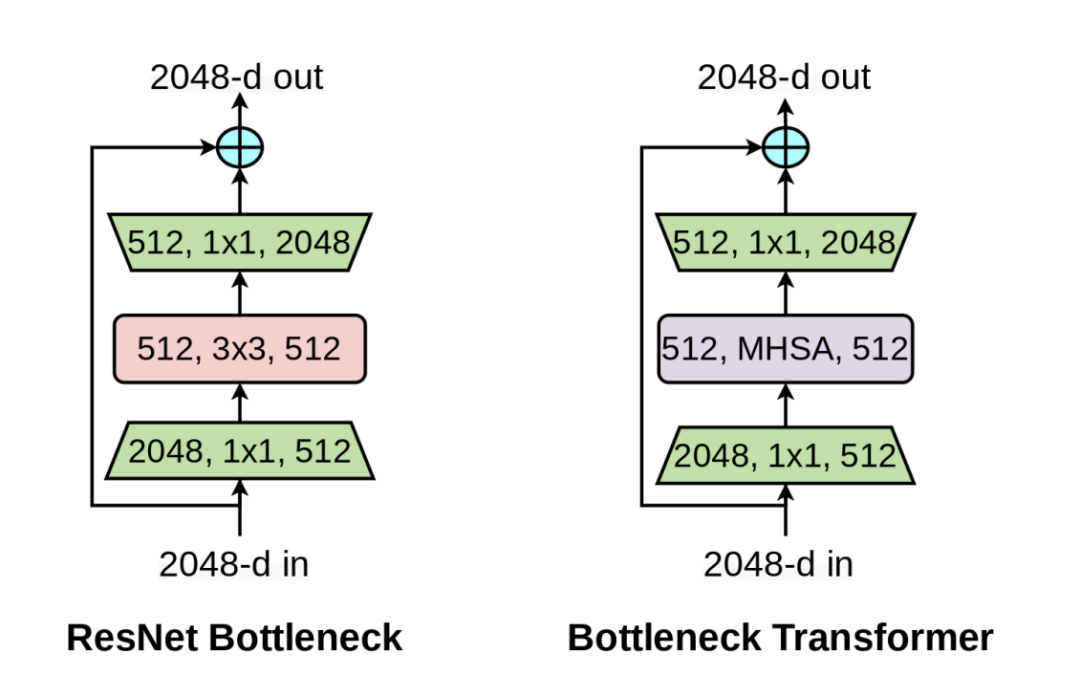Bot模块图

BotNet作为Backbone

``     if x and y:            self.branch2b = paddle.nn.LayerList()            self.branch2b.append(MHSA(512, width=int(38),                             height=int(38), heads=4))            self.branch2b.append(paddle.nn.AvgPool2D(2, 2))            self.branch2b = paddle.nn.Sequential(*self.branch2b)        elif x and not y:            self.branch2b = MHSA(512,width=19, height=19)        else:                self.branch2b = ConvNormLayer(            ch_in=width,            ch_out=width,            filter_size=3,            stride=stride2,            groups=groups,            act='relu',            norm_type=norm_type,            norm_decay=norm_decay,            freeze_norm=freeze_norm,            lr=lr,            dcn_v2=dcn_v2)``

https://aistudio.baidu.com/aistudio/projectdetail/2265776

AI Studio主页：

https://aistudio.baidu.com/aistudio/personalcenter/thirdview/553083

Bottleneck Transformers for Visual Recognition：https://arxiv.org/abs/2101.11605

Star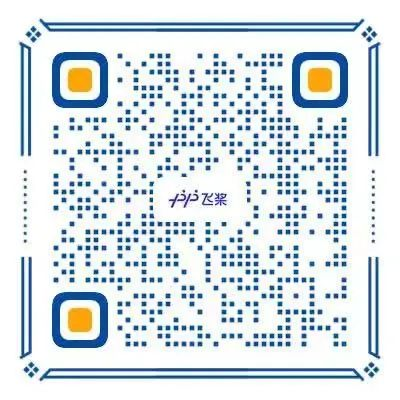1. 飞桨官方QQ群：793866180

2. 飞桨官网网址：

3. 飞桨开源框架项目地址：

GitHub: